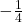## What is the equation of the quadratic graph with a focus of (3, -1) and a directrix of y = 1?

Question

What is the equation of the quadratic graph with a focus of (3, -1) and a directrix of y = 1?

in progress 0
6 months 2021-07-29T20:33:44+00:00 1 Answers 7 views 0

## Answers ( )

The equation of the quadratic graph is y =(x – 3)²

Step-by-step explanation:

The standard form of the equation of the quadratic graph is

(x – h)² = 4p(y – k)
, where

• The vertex of the parabola is (h, k)
• The focus is (h, k + p)
• The directrix is at y = k – p

∵ The focus is (3, -1)

∵ The focus is (h, k + p)

→ Compare them

h = 3

k + p = -1 ⇒ (1)

∵ The directrix is at y = 1

∵ The directrix is at y = k – p

→ Compare them

k – p = 1 ⇒ (2)

→ Add equations (1) and (2) to eliminate p

∵ (k + k) + (p – p) = (-1 + 1)

∴ 2k + 0 = 0

∴ 2k = 0

→ Divide both sides by 2

K = 0

→ Substitute the value of k in equation (1) to find p

∵ 0 + p = -1

p = -1

→ Substitute the values of h, k, and p in the form of the equation above

(x – 3)² = 4(-1)(y – 0)

∴ (x – 3)² = -4(y)

(x – 3)² = -4y

→ Divide both sides by -4(x – 3)² = y

→ Switch the two sides

y =(x – 3)²

The equation of the quadratic graph is y =(x – 3)²